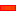﻿ Step 15.7: Index the Belt

# Step 15.7: Index the Belt

### Background:

The Belt is modelled to move with constant velocity.  We want it to index.

An Indexing Belt is one where, in one machine cycle:

 1 There is an Index Period: The belt moves to a position during one phase of the machine cycle.
 2 There is a Dwell Period: The belt stops at that position for the remainder of the machine cycle.

We must change the motion-values that we give to the kinematic-chains.

We connect new Function-Blocks to the input-connector of the Motion-Dimension FB to give a different motion to the Belt.

####Function-Blocks for a Constant Velocity BeltSchematic of FBs of one Slider Set for belt moving at constant velocity. Schematic of the Function-Blocks for a Constant-Velocity Belt The image to the left is a schematic of how to connect the Function-Blocks to model the Path of the Constant Velocity Belt. The MMA motion value is the independent variable at the input of the separate X & Y Motion FBs. For each motion value at the input of a Motion FB, there is a specified motion value at the output. It does not matter how fast or slow the input changes (to change the speed of the Belt), the motion value at each output-connector is the same for each motion-value at a input-connector. The MMA increases continuously from 0 to 360, and again and again. The X & Y Motions were specified to give a constant velocity Belt. See Design the Motion for the Belt.####Function-Blocks for an Indexing BeltSchematic of one Slider Set for belt moving at constant velocity.

Schematic of the Function-Blocks for an Indexing Belt

We want the belt to index along the path, and stop at fixed positions during the dwell.

To do this, replace the motion that is given to the input-connectors of the Motion FBs

 • We replace the steady motion, provided by the MMA...
 • ...with an Indexing Motion linked to a Motion FB

Note: the MMA must still be at the input-connector to the Indexing Motion FBThis is an example 'Indexing Motion'.

Segment 1: Output increases to 360º, as the input changes from 0º to 180º, the output motion indexes [increases] to 360, also.

Segment 2: Output is a Dwell, as the input changes from 180º to 360º

The image shows the position and acceleration graphs.Question: Spot the problem?

Answer: The output from the indexing motion changes from 0 – 360º during a machine cycle.

Thus the belt and pocket moves all the way around from the start position back to the start position again.

It does this in one machine cycle [0 – 360 of the MMA].

We need to do two things:

 • Index the Belt 12 times, not one time, all within one machine cycle [0 – 360 of the MMA].

- and -

 • Index the Belt 30º, not 360. This is one pocket, not 12 Pockets, each index.Index the Belt 12 times...

To do the indexing motion twelve times in one machine cycle [0 – 360 of the MMA], connect a Gearing FBto the input to the Indexing Motion. Edit the Gearing Ratio parameter to 12.

Add a Gearing FB. Edit it, and enter 12 as the Gearing Ratio parameter.

Now, the motion-values at the output from the Gearing FB, and thus also the input to the Motion FB, are from 0 to 4320º each time the MMA changes from 0 to 360.Index the Belt 30º...

To index the Belt 30º, edit the Motion in MotionDesigner.

The Motion Design to the left:

Segment 1: Y-axis changes from 0 to 30º , as the X-axis changes from 0º to 180º

Segment 2: Y-axis is at 30º, [dwells], as the X-axis changes from 180º to 360º.

It does a total of 12 indexes, in one machine cycle [MMA], because the input to the Motion FB changes from 0 to 4320.The Graph to the left shows the output from the Motion FB as a function of its input, which is now 0 – 4320º

Question: Spot the Problem?

Answer:  The output from the Motion FB increase to 30º after each index motion, but immediately resets to 0º. It repeats 12 times.We will use the 'Accumulate Output radio-button' in the Motion FB dialog-box.

 1 Open the Motion FB again
 2 Select the 'Accumulate Output' radio button.This graph shows:

 • Y-axis: output from the Motion FB, which has a from 0º to 360º.
 • X-axis: input to the Motion FB [or the output from the Gearing FB], which has a range from 0º to 4320º.

The output [Y-axis] from the Motion FB increases from 0 – 30º for the first 180º of the of the, and stays at 30º between 180 – 360º.

Then the output [Y-axis]t increases to 60º for from 360º to 540º

There are 12 indexes in one cycle of the MMA. Each index increases the position of the output by 30º.

This is the motion that we want for the pocket and chain-link

The pocket and chain-link will move 12 times, in one cycle of the MMA.

Tutorial and Reference Help Files for MechDesigner and MotionDesigner 13.2 + © Machine, Mechanism, Motion and Cam Design Software by PSMotion Ltd